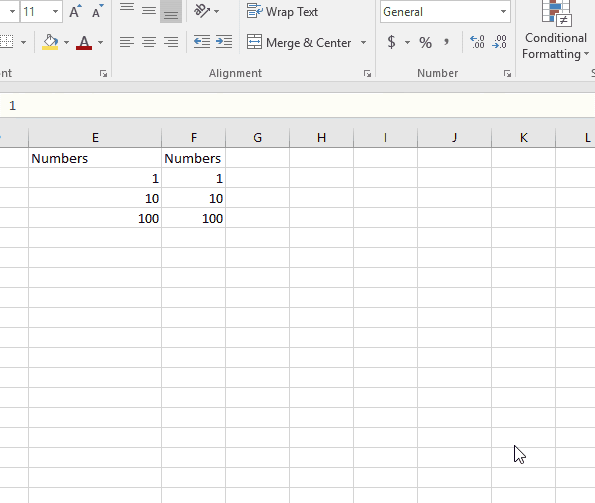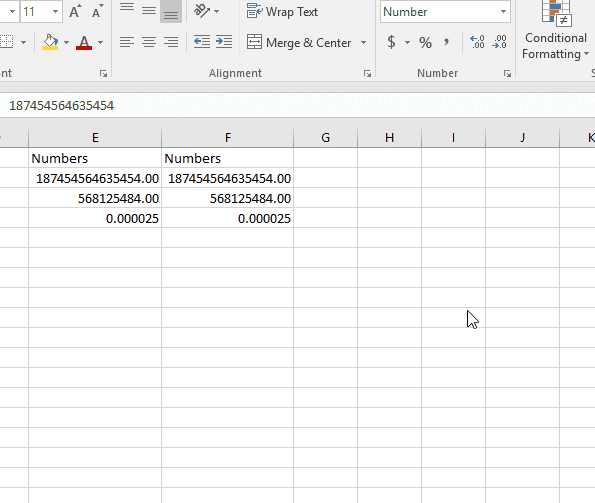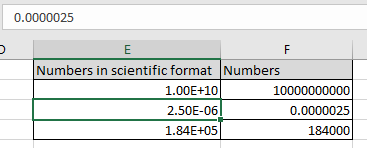# How to use Scientific formatting in ExcelIn this article, we will learn How to use Scientific formatting in Excel.

Number formatting in Excel

The Scientific format displays a number in exponential notation, replacing part of the number with E+n, in which E (exponent) multiplies the preceding number by 10 to the nth power. Number can be changed to percentage, date, time, currency and scientific number.

In simple words, sometimes working with mathematical operations on a sheet. We need numbers in Scientific format. Excel allows us to convert the format of the cell to scientific format.

For example, a 2-decimal scientific format displays 12345678901 as 1.23E+10, which is 1.23 times 10 to the 10th power.

Example :

All of these might be confusing to understand. Let's understand how to use the function using an example. Here we have 3 multiples of 10. Let’s see how to convert them into scientific format.As you can see, we can convert numbers from general to scientific format.You can convert multiples to 10 and decimal values to scientific format. When you convert a decimal value to scientific format, the number gets displayed in E-n format.

Now we will understand how to write in scientific format

Write 10^10 in scientific :

 1e+10

It will be displayed as 1.00E+10 in the cell.

Write 0.0000025 in scientific :

 2.5-6

It will be displayed as 2.5-06 in the cell.

Write 184000 in scientific :

 184000

It will be displayed as 1.84E+05 in the cell.

The above 3 representation of scientific format of numbers, shown in the snapshot below.Here cells have been converted to scientific format & written in Excel.

Here are all the observational notes using the number formatting in Excel.
Notes :

1. Use number formatting to convert the value into another format. The value remains the same.
2. Use percentage format when using percentage rate values in Excel.
3. Formula starts with ( = ) sign.
4. Sometimes there exist a curly braces in the start of formula, which is an array formula and called using the Ctrl + Shift + Enter. Do not put curly braces manually.
5. Text values and characters are always given using quotes or using cell reference
6. Numerical values can be given directly or using the cell reference.
7. Array can be given using the array reference or using named ranges.

Hope this article about How to use Scientific formatting in Excel is explanatory. Find more articles on calculating values and related Excel formulas here. If you liked our blogs, share it with your friends on Facebook. And also you can follow us on Twitter and Facebook. We would love to hear from you, do let us know how we can improve, complement or innovate our work and make it better for you. Write to us at info@exceltip.com.

Related Articles :

Evaluation of Excel Formula Step By Step : Excel provides functionality that helps us evaluate the formula. We can see how a formula is working by stepping through the formula.

How to use the Shortcut To Toggle Between Absolute and Relative References in Excel : F4 shortcut to convert absolute to relative reference and same shortcut use for vice versa in Excel.

How to use Shortcut Keys for Merge and Center in Excel : Use Alt and then follow h, m and c to Merge and centre cells in Excel.

How to Select Entire Column and Row Using Keyboard Shortcuts in Excel : Use Ctrl + Space to select whole column and  Shift + Space to select whole row using keyboard shortcut in Excel

Paste Special Shortcut in Mac and Windows : In windows, the keyboard shortcut for paste special is Ctrl + Alt + V. Whereas in Mac, use Ctrl + COMMAND + V key combination to open the paste special dialog in Excel.

How to Insert Row Shortcut in Excel : Use Ctrl + Shift + = to open the Insert dialog box where you can insert row, column or cells in Excel.

Excel REPLACE vs SUBSTITUTE function: The REPLACE and SUBSTITUTE functions are the most misunderstood functions. To find and replace a given text we use the SUBSTITUTE function. Where REPLACE is used to replace a number of characters in string…

Popular Articles :

How to use the IF Function in Excel : The IF statement in Excel checks the condition and returns a specific value if the condition is TRUE or returns another specific value if FALSE.

How to use the VLOOKUP Function in Excel : This is one of the most used and popular functions of excel that is used to lookup value from different ranges and sheets.

How to use the SUMIF Function in Excel : This is another dashboard essential function. This helps you sum up values on specific conditions.

How to use the COUNTIF Function in Excel : Count values with conditions using this amazing function. You don't need to filter your data to count specific values. Countif function is essential to prepare your dashboard.

Terms and Conditions of use

The applications/code on this site are distributed as is and without warranties or liability. In no event shall the owner of the copyrights, or the authors of the applications/code be liable for any loss of profit, any problems or any damage resulting from the use or evaluation of the applications/code.